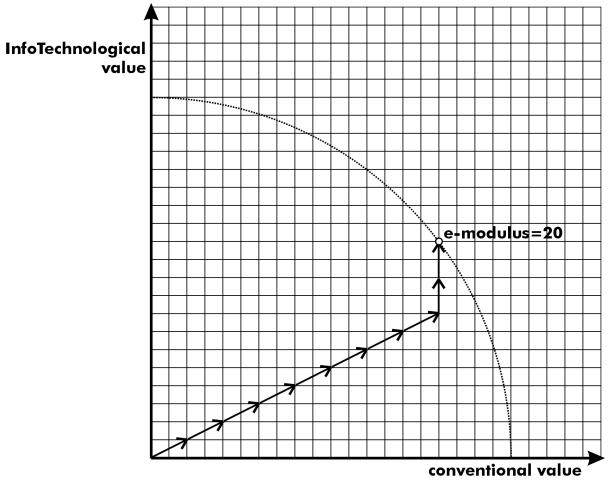Online JudgeProblem SetAuthorsOnline ContestsUser
Web Board
F.A.Qs
Statistical Charts
Problems
Submit Problem
Online Status
Prob.ID:
Register
Authors ranklist
Current Contest
Past Contests
Scheduled Contests
Award Contest
Register
Language:
e-Coins
 Time Limit: 1000MS Memory Limit: 65536K Total Submissions: 879 Accepted: 426

Description

At the Department for Bills and Coins, an extension of today's monetary system has newly been proposed, in order to make it fit the new economy better. A number of new so called e-coins will be produced, which, in addition to having a value in the normal sense of today, also have an InfoTechnological value. The goal of this reform is, of course, to make justice to the economy of numerous dotcom companies which, despite the fact that they are low on money surely have a lot of IT inside. All money of the old kind will keep its conventional value and get zero InfoTechnological value.

To successfully make value comparisions in the new system, something called the e-modulus is introduced. This is calculated as SQRT(X*X+Y*Y), where X and Y hold the sums of the conventional and InfoTechnological values respectively. For instance, money with a conventional value of \$3 altogether and an InfoTechnological value of \$4 will get an e-modulus of \$5. Bear in mind that you have to calculate the sums of the conventional and InfoTechnological values separately before you calculate the e-modulus of the money.

To simplify the move to e-currency, you are assigned to write a program that, given the e-modulus that shall be reached and a list of the different types of e-coins that are available, calculates the smallest amount of e-coins that are needed to exactly match the e-modulus. There is no limit on how many e-coins of each type that may be used to match the given e-modulus.

Input

A line with the number of problems n (0 < n<=100), followed by n times:
• A line with the integers m (0 < m<=40) and S (0 < S<=300), where m indicates the number of different e-coin types that exist in the problem, and S states the value of the e-modulus that shall be matched exactly.
• m lines, each consisting of one pair of non-negative integers describing the value of an e-coin. The first number in the pair states the conventional value, and the second number holds the InfoTechnological value of the coin.
When more than one number are present on a line, they will be separated by a space. Between each problem, there will be one blank line.

Output

The output consists of n lines. Each line contains either a single integer holding the number of coins necessary to reach the specified e-modulus S or, if S cannot be reached, the string "not possible".

Sample Input

```3
2 5
0 2
2 0

3 20
0 2
2 0
2 1

3 5
3 0
0 4
5 5
```

Sample Output

```not possible
10
2```

HintThe illustration examplifies adding 8 coins of conventional value 2 and InfoTechnological value 1, and 2 coins with pure InfoTechnological value 2. The e-modulus is of course 20 as SQRT((8*2+2*0)^2+(8*1+2*2)^2)=SQRT(16^2+12^2)=20

Source

[Submit]   [Go Back]   [Status]   [Discuss]Home PageGo BackTo top